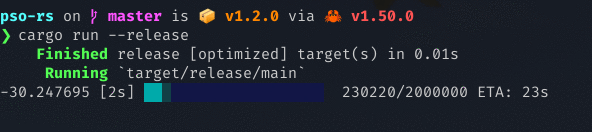# Crate pso_rs[−][src]

Expand description

An easy-to-use, simple Particle Swarm Optimization (PSO) implementation in Rust.

It uses the `rand` crate for random initialization, and the `rayon` crate for parallel objective function computation. It also has a nice progress bar curtesy of the `indicatif` crate. Below is a screenshot of PSO running, attempting to minimize the Lennard-Jones potential energy in a cluster of 20 molecules:The examples below can get you started. In order to use it in your own optimization problem, you will need to define an objective function as it is defined in the run function, and a `Config` object. See the Notes section for more tips.

## Examples

### Run PSO

``````use pso_rs::*;

// define objective function (d-dimensional Rosenbrock)
fn objective_function(
p: &Particle,
_flat_dim: usize,
dimensions: &Vec<usize>
) -> f64 {
(0..dimensions - 1).map(|i| {
100.0 * ((p[i+1]-p[i]).powf(2.0)).powf(2.0)
+ (1.0-p[i]).powf(2.0)
}).sum()
}

// define a termination condition (optional)
fn terminate(f_best: f64) -> bool {
f_best - (0.0) < 1e-4
}

let config = Config {
// dimension shape of each particle
dimensions: vec!,
// problem bounds in each dimension
bounds: vec![(-5.0, 10.0); 2],
// maximum no. of objective function computations
t_max: 10000,
// leave the rest of the params as default
..Config::default()
};

let pso = pso_rs::run(
config,
objective_function,
Some(terminate)
).unwrap();

let model = pso.model;
println!("Model: {:?} ", model.get_f_best());``````

### Initialize PSO for later execution

``````use pso_rs::*;

// define objective function (d-dimensional Rosenbrock)
fn objective_function(
p: &Particle,
_flat_dim: usize,
dimensions: &Vec<usize>
) -> f64 {
(0..dimensions - 1).map(|i| {
100.0 * ((p[i+1]-p[i]).powf(2.0)).powf(2.0)
+ (1.0-p[i]).powf(2.0)
}).sum()
}

let config = Config {
dimensions: vec!,
bounds: vec![(-5.0, 10.0); 2],
t_max: 10000,
..Config::default()
};

let mut pso = pso_rs::init(
config,
objective_function
).unwrap();

// run PSO with no termination condition
pso.run(|_| false);

let model = pso.model;
println!("Found minimum: {:#?} ", model.get_f_best());
println!("Minimizer: {:#?}", model.get_x_best());``````

### Performance

This implementation uses a flat vector (`Vec<f64>`) to represent any d-dimensional problem (see the Optimization Problem Dimensionality section). This means that the vector has an O(1) access time, and can be cached for fast access, similarly to a static array.

The computation of the objective function for each particle is performed in parallel, as it is computationally expensive for any non-trivial problem. In the future, complete swarms will be able to be run in parallel and optionally communicate their best found positions by passing messages.

### Optimization problem dimensionality

Even though you can have particles of any shape and size, as long as each item is `f64`, `pso_rs` represents each particle as a flat vector: `Vec<f64>`.

This means that, for example, in order to find clusters of 20 molecules in 3D space that minimize the Lennard-Jones potential energy, you can define `dimensions` as (20, 3). If you want, you can also create a custom `reshape` function, like this one for molecule clusters below:

``````use pso_rs::*;

fn reshape(
particle: &Particle,
particle_dims: &Vec<usize>
) -> Vec<Vec<f64>> {
let mut reshaped_cluster = vec![];
let mut i = 0;
for _ in 0..particle_dims {
let mut reshaped_molecule = vec![];
for _ in 0..particle_dims {
reshaped_molecule.push(particle[i]);
i += 1;
}
reshaped_cluster.push(reshaped_molecule);
}
reshaped_cluster
}

// used in the objective function
fn objective_function(
p: &Particle,
_flat_dim: usize,
dimensions: &Vec<usize>
) -> f64 {
let _reshaped_particle = reshape(p, dimensions);
/* Do stuff */
0.0
}

let config = Config {
dimensions: vec![20, 3],
bounds: vec![(-2.5, 2.5); 3],
t_max: 1,
..Config::default()
};

let pso = pso_rs::run(
config,
objective_function,
None
).unwrap();

// somewhere in main(), after running PSO as in the example:
println!(
"Best found minimizer: {:#?} ",
reshape(&pso.model.get_x_best(),
&pso.model.config.dimensions)
);``````

## Re-exports

`pub use model::*;`

## Functions

Initializes and returns a PSO instance without running the optimization process

Creates a model and runs the PSO method